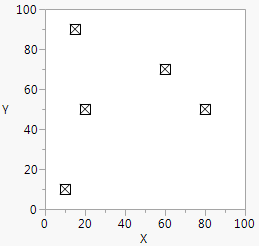Publication date: 11/10/2021

## Draw with Pixels

You can also draw using pixel coordinates. First, set the Pixel Origin() in terms of graph coordinates, and then use Pixel Move To() or Pixel Line To() in pixel coordinates relative to that origin. The main use for Pixel() functions is for drawing custom markers that do not vary with the size or scale of the graph. You can store a marker in a script and then call it within any graph. This example uses Function() to store pixel commands in a script with its own arguments, x and y.

`ballotBox = Function( {x, y},`
`	Pixel Origin( x, y );`
`	Pixel Move To( -5, -5 );`
`	Pixel Line To( -5, 5 );`
`	Pixel Line To( 5, -5 );`
`	Pixel Line To( -5, -5 );`
`	Pixel Line To( 5, 5 );`
`	Pixel Line To( -5, 5 );`
`	Pixel Move To( 5, 5 );`
`	Pixel Line To( 5, -5 );`
`);`
`win = New Window( "Custom Markers",`
`	Graph Box(`
`		Frame Size( 200, 200 ),`
`		ballotBox( 10, 10 );`
`		ballotBox( 15, 90 );`
`		ballotBox( 20, 50 );`
`		ballotBox( 80, 50 );`
`		ballotBox( 60, 70 );`
`	)`
`);`

Figure 12.22 Drawing Custom Markers